# JP2010263953A - Motion analysis device, program and method thereof, and motion analysis system - Google Patents

Motion analysis device, program and method thereof, and motion analysis system Download PDF

## Info

Publication number
JP2010263953A
JP2010263953A JP2009115720A JP2009115720A JP2010263953A JP 2010263953 A JP2010263953 A JP 2010263953A JP 2009115720 A JP2009115720 A JP 2009115720A JP 2009115720 A JP2009115720 A JP 2009115720A JP 2010263953 A JP2010263953 A JP 2010263953A
Authority
JP
Japan
Prior art keywords
motion
analysis
exercise
predetermined
frequency
Prior art date
Legal status (The legal status is an assumption and is not a legal conclusion. Google has not performed a legal analysis and makes no representation as to the accuracy of the status listed.)
Pending
Application number
JP2009115720A
Other languages
Japanese (ja)
Inventor
Kurato Maeno
Daisuke Okutani

Original Assignee
Oki Electric Ind Co Ltd

Priority date (The priority date is an assumption and is not a legal conclusion. Google has not performed a legal analysis and makes no representation as to the accuracy of the date listed.)
Filing date
Publication date
Application filed by Oki Electric Ind Co Ltd, 沖電気工業株式会社 filed Critical Oki Electric Ind Co Ltd
Priority to JP2009115720A priority Critical patent/JP2010263953A/en
Publication of JP2010263953A publication Critical patent/JP2010263953A/en
Application status is Pending legal-status Critical

• 238000004458 analytical methods Methods 0 abstract title 5
• 230000003595 spectral Effects 0 abstract 2
• 241000282414 Homo sapiens Species 0 abstract 1

## Images

•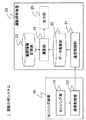•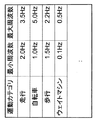•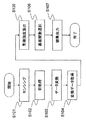•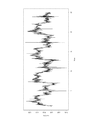•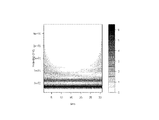•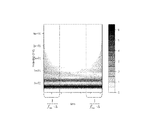•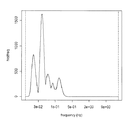•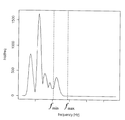•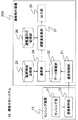•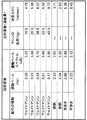•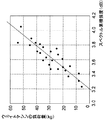•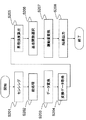•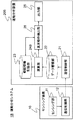•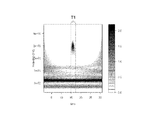•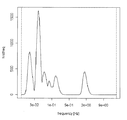•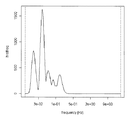•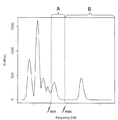•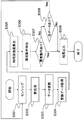## Classifications

• AHUMAN NECESSITIES
• A61MEDICAL OR VETERINARY SCIENCE; HYGIENE
• A61BDIAGNOSIS; SURGERY; IDENTIFICATION
• A61B5/00Detecting, measuring or recording for diagnostic purposes; Identification of persons
• A61B5/103Detecting, measuring or recording devices for testing the shape, pattern, colour, size or movement of the body or parts thereof, for diagnostic purposes
• A61B5/11Measuring movement of the entire body or parts thereof, e.g. head or hand tremor, mobility of a limb
• A61B5/1123Discriminating type of movement, e.g. walking or running
• AHUMAN NECESSITIES
• A61MEDICAL OR VETERINARY SCIENCE; HYGIENE
• A61BDIAGNOSIS; SURGERY; IDENTIFICATION
• A61B5/00Detecting, measuring or recording for diagnostic purposes; Identification of persons
• A61B5/103Detecting, measuring or recording devices for testing the shape, pattern, colour, size or movement of the body or parts thereof, for diagnostic purposes
• A61B5/1036Measuring load distribution, e.g. podologic studies
• A61B5/1038Measuring plantar pressure during gait
• AHUMAN NECESSITIES
• A63SPORTS; GAMES; AMUSEMENTS
• A63BAPPARATUS FOR PHYSICAL TRAINING, GYMNASTICS, SWIMMING, CLIMBING, OR FENCING; BALL GAMES; TRAINING EQUIPMENT
• A63B24/00Electric or electronic controls for exercising apparatus of preceding groups; Controlling or monitoring of exercises, sportive games, training or athletic performances
• A63B24/0062Monitoring athletic performances, e.g. for determining the work of a user on an exercise apparatus, the completed jogging or cycling distance
• AHUMAN NECESSITIES
• A61MEDICAL OR VETERINARY SCIENCE; HYGIENE
• A61BDIAGNOSIS; SURGERY; IDENTIFICATION
• A61B2562/00Details of sensors; Constructional details of sensor housings or probes; Accessories for sensors
• A61B2562/02Details of sensors specially adapted for in-vivo measurements
• A61B2562/0219Inertial sensors, e.g. accelerometers, gyroscopes, tilt switches
• AHUMAN NECESSITIES
• A61MEDICAL OR VETERINARY SCIENCE; HYGIENE
• A61BDIAGNOSIS; SURGERY; IDENTIFICATION
• A61B5/00Detecting, measuring or recording for diagnostic purposes; Identification of persons
• A61B5/72Signal processing specially adapted for physiological signals or for diagnostic purposes
• A61B5/7235Details of waveform analysis
• A61B5/7253Details of waveform analysis characterised by using transforms
• A61B5/726Details of waveform analysis characterised by using transforms using Wavelet transforms
• AHUMAN NECESSITIES
• A63SPORTS; GAMES; AMUSEMENTS
• A63BAPPARATUS FOR PHYSICAL TRAINING, GYMNASTICS, SWIMMING, CLIMBING, OR FENCING; BALL GAMES; TRAINING EQUIPMENT
• A63B24/00Electric or electronic controls for exercising apparatus of preceding groups; Controlling or monitoring of exercises, sportive games, training or athletic performances
• A63B24/0062Monitoring athletic performances, e.g. for determining the work of a user on an exercise apparatus, the completed jogging or cycling distance
• A63B2024/0065Evaluating the fitness, e.g. fitness level or fitness index
• AHUMAN NECESSITIES
• A63SPORTS; GAMES; AMUSEMENTS
• A63BAPPARATUS FOR PHYSICAL TRAINING, GYMNASTICS, SWIMMING, CLIMBING, OR FENCING; BALL GAMES; TRAINING EQUIPMENT
• A63B24/00Electric or electronic controls for exercising apparatus of preceding groups; Controlling or monitoring of exercises, sportive games, training or athletic performances
• A63B24/0062Monitoring athletic performances, e.g. for determining the work of a user on an exercise apparatus, the completed jogging or cycling distance
• A63B2024/0071Distinction between different activities, movements, or kind of sports performed
• AHUMAN NECESSITIES
• A63SPORTS; GAMES; AMUSEMENTS
• A63BAPPARATUS FOR PHYSICAL TRAINING, GYMNASTICS, SWIMMING, CLIMBING, OR FENCING; BALL GAMES; TRAINING EQUIPMENT
• A63B2220/00Measuring of physical parameters relating to sporting activity
• A63B2220/40Acceleration
• AHUMAN NECESSITIES
• A63SPORTS; GAMES; AMUSEMENTS
• A63BAPPARATUS FOR PHYSICAL TRAINING, GYMNASTICS, SWIMMING, CLIMBING, OR FENCING; BALL GAMES; TRAINING EQUIPMENT
• A63B2220/00Measuring of physical parameters relating to sporting activity
• A63B2220/80Special sensors, transducers or devices therefor
• A63B2220/803Motion sensors
• AHUMAN NECESSITIES
• A63SPORTS; GAMES; AMUSEMENTS
• A63BAPPARATUS FOR PHYSICAL TRAINING, GYMNASTICS, SWIMMING, CLIMBING, OR FENCING; BALL GAMES; TRAINING EQUIPMENT
• A63B2230/00Measuring physiological parameters of the user
• A63B2230/75Measuring physiological parameters of the user calorie expenditure

## Abstract

PROBLEM TO BE SOLVED: To perform a highly accurate analysis with a small amount of processing in an analysis related to a motion of an object such as a person or an object.
The present invention provides a motion comprising a sensor device that measures the amount of motion related to an object that repeatedly performs a predetermined motion, and a motion analysis device that performs analysis related to the predetermined motion of the object based on the measurement result of the sensor device. It relates to an analysis system. Then, the motion analysis device uses a predetermined function for the time series data of the momentum measured by the sensor device and the waveform indicated by the stored time series data, and analyzes the spectrum intensity for each time series for each frequency. Comparing between the different function for each frequency to the above function and analyzing the spectrum intensity analysis result for each frequency between different frequencies, and based on the comparison result, And a means for analyzing a predetermined physical quantity related to the movement of the robot.
[Selection] Figure 1

## Description

The present invention relates to a motion analysis apparatus, a program and a method, and a motion analysis system, and can be applied to, for example, a system for analyzing the pace of motion of an object such as a person or an object that repeatedly performs a predetermined motion.

2. Description of the Related Art Conventionally, a method for detecting the pace and amount of exercise by attaching an exercise sensor to a human body in order to recognize and use a person's condition such as health condition management and rehabilitation has been widely devised.

In Patent Documents 1 to 4, acceleration sensors are attached to places such as the waist, legs, and arms, and a waveform resulting from a temporal change in acceleration obtained during walking motion is converted into a frequency component by Fourier transform, resulting in a peak in spectral intensity. If the sensor is attached to the waist, two steps will be one cycle, and if the sensor is attached to the leg, one step will be one cycle. The pace of exercise is calculated.

In Patent Documents 5 and 6 and Patent Document 7, paying attention to the shape of the waveform for each motion period, an index indicating the pace of the length of one motion period using a method using a peak value interval or a zero cross point is used. It describes the technology to be acquired.

JP 2006-118909 A JP 2005-233771 A JP 2005-342254 A JP 2004-121539 A JP 2005-49202 A JP 2008-154733 A JP 2006-101973 A

However, all of the above-mentioned Patent Documents 1 to 7 deal with walking with a single exercise cycle of about 1 Hz to 3.5 Hz as an object of exercise, for example, training with a dumbbell or using a weight machine in a gym. It is difficult to apply to a motion that is longer in one cycle than a walking motion, such as lifting an object or operating a device in daily life, or a motion in which the shape of an acceleration waveform in one cycle is complicated. When it is intended to manage the human condition such as health condition management, rehabilitation, and the work contents of the worker, it is necessary to handle exercises other than walking movements widely.

In the methods based on the Fourier transform including the techniques described in Patent Documents 1 to 4, the range of the obtained pace frequency is up to ½ (Nyquist frequency) of the sampling rate at which the sensor acquires data, and the resolution is the sensor. Depends on the sampling rate at which the data is acquired and the time window to which the Fourier transform is applied. In order to increase the resolution of the pace, it is necessary to decrease the sampling rate of the sensor or increase the time window of the Fourier transform.

In general, the walking pace is not relatively low compared with the sampling rate of the sensor, and the difference in walking pace can be sufficiently discriminated with a resolution using a method based on Fourier transform. However, in order to identify the pace of movement slower than walking with high resolution, it is necessary to lower the sampling rate of the sensor or to repeat one movement for a long time. Lowering the sampling rate truncates exercise information, and repeating the same exercise for a long time cannot be a realistic solution.

Further, even if the movement is slower than the walking movement, there are a wide variety of movement types, and the waveforms obtained by the sensors in each movement are greatly different in amplitude, period, and shape.

In the method using the waveform shape obtained from the sensors as in Patent Documents 5 to 7, it is necessary to accurately determine the waveform for one cycle of motion, and the waveform representing motion such as reduction of noise components using a low-pass filter. Preprocessing to make the shape of the image stand out plays an important role, but it is not easy to obtain an appropriate peak value and zero cross point from a complex waveform without preprocessing. Therefore, it is not easy to obtain a pace by collectively performing various exercises.

Therefore, there is a demand for a motion analysis apparatus, program and method, and motion analysis system that can perform highly accurate analysis with a small amount of processing in analysis related to motion of an object such as a person or an object.

The first motion analysis device includes: (1) a sensor device that measures a momentum related to a motion of an object that repeatedly performs the predetermined motion of the present invention; and the predetermined motion of the object based on a measurement result of the sensor device. (2) Time series data holding means for holding time series data on the amount of exercise measured by the sensor device; and (3) Applying a predetermined function to the waveform indicated by the time series data to analyze the spectrum intensity for each frequency, and applying a parameter different for each frequency to the function to analyze the waveform (4) The spectral intensity analysis result for each frequency analyzed by the waveform analysis means is compared between different frequencies, and the object is determined based on the comparison result. And having a motion analysis means for analyzing a certain physical amount of the certain motion definitive.

The motion analysis program of the second aspect of the present invention includes (1) a sensor device that measures a momentum related to a motion of an object that repeatedly performs a predetermined motion, and the predetermined motion of the object based on a measurement result of the sensor device. (2) time series data holding means for holding the time series data of the amount of exercise measured by the sensor device; And (3) applying a predetermined function to the waveform indicated by the time series data to analyze the spectrum intensity for each frequency, and applying a different parameter for each frequency to the function for analysis. And (4) comparing the spectral intensity analysis results for each frequency analyzed by the waveform analyzing means between different frequencies, and comparing the results. Based on the result, characterized in that to function as motion analysis means for analyzing a certain physical amount of the certain motion of the object.

The motion analysis method of the third aspect of the present invention is (1) performing an analysis related to the predetermined motion in the object based on a measurement result of a sensor device that measures a momentum related to the motion of the object that repeatedly performs the predetermined motion. In the motion analysis method, (2) time series data holding means, waveform analysis means, and motion analysis means are provided. (3) The time series data holding means holds time series data of the momentum measured by the sensor device. (4) the waveform analysis means applies a predetermined function to the waveform indicated by the time series data and analyzes the spectrum intensity for each frequency. (5) The motion analysis means analyzes the spectrum intensity analysis result for each frequency analyzed by the waveform analysis means with different frequencies. In comparison, based on the comparison result, characterized by analyzing the predetermined physical amount of the certain motion of the object.

A motion analysis system according to a fourth aspect of the present invention includes (1) a sensor device that measures a momentum related to a motion of an object that repeatedly performs a predetermined motion, and the predetermined motion of the object based on a measurement result of the sensor device. A motion analysis system including the motion analysis device that performs the analysis according to (2) is characterized in that the motion analysis device according to the first aspect of the present invention is applied as the motion analysis device.

According to the present invention, it is possible to perform a highly accurate analysis with a small amount of processing in an analysis related to the movement of an object such as a person or an object.

(A) First Embodiment Hereinafter, a motion analysis device, a program and method, and a motion analysis system according to a first embodiment of the present invention will be described in detail with reference to the drawings.

(A-1) Configuration of the First Embodiment FIG. 1 is a block diagram showing the overall configuration of the motion analysis system 1 of the first embodiment.

The motion analysis system 1 includes a sensing device 10 and a motion analysis device 20.

The sensing device 10 is a device for measuring an amount (hereinafter referred to as “exercise amount”) relating to an exercise of a user who performs a predetermined exercise (hereinafter referred to as “exercise user”), and a sensing unit 11 that measures the amount of exercise, It has the communication control part 12 which transmits a measurement result to the motion analysis apparatus 20. FIG. In the first embodiment, the motion to be analyzed in the motion analysis system 1 is a motion of a motion user who is a human. However, the sensing device 10 may be a non-human animal or object (for example, an automobile). It may be attached to another object such as a vehicle or a machine tool, and the motion of the object may be analyzed, and the object to be analyzed in the motion analysis system 1 is not limited. In the motion analysis system 1, the type of motion to be analyzed is not limited as long as it is a motion that is repeatedly performed to some extent, such as a weight machine (exercise using the weight machine) and walking.

Although it is desirable to attach the sensing device 10 to a part with a large fluctuation in motion according to the category of exercise of the exercise user, such as an upper arm, a wrist, or a waist, for example, the attachment position is not particularly limited. In addition, the sensing device 10 may be fixed to the exercise user with a tape or a belt, or may be put in the pocket of the exercise user, and the method for attaching to the exercise user is not limited. Then, when the exercise user performs a predetermined exercise with the sensing device 10 attached to the exercise user, the sensing device 10 itself exercises with the exercise user's exercise. The amount of exercise can be regarded as the amount of exercise of the exercise user. In the following description, the sensing device 10 measures its own acceleration as a momentum, but other physical quantities such as a movement amount and a movement speed per unit time may be applied.

The sensing unit 11 has a function of measuring the exercise amount of the exercise user and transmitting data of the measurement result (hereinafter referred to as “exercise data”) to the exercise analysis device 20 via the communication control unit 12. The sensing unit 11 measures the acceleration accompanying the exercise of the exercise user for each predetermined cycle (hereinafter referred to as “sampling cycle”) using an existing acceleration sensor or the like. The sensing unit 11 measures acceleration regardless of the sampling period, and extracts motion data for each time series on the motion analyzer 20 side to form time series data (hereinafter referred to as “time series motion data”). You may do it. In the following description, the sensing unit 11 is described as applying a triaxial acceleration sensor, but a sensor capable of observing temporal changes by movement, such as an angular velocity sensor, a myoelectric potential sensor, a tilt sensor, a Doppler radar, You can use motion capture from video, or 1-axis and 2-axis acceleration sensors, and attach sensors to multiple locations to integrate and analyze the values of each sensor or select values with large characteristics. May be used.

The communication control unit 12 functions as an interface for communicating with the motion analysis device 20, and transmits the motion data given from the sensing unit 11 to the motion analysis device 20.

The exercise analysis device 20 acquires exercise data from the sensing device 10, analyzes the exercise data, and performs analysis related to the exercise performed by the exercise user. The communication control unit 21, the data storage unit 22, range information A storage unit 23, a calculation unit 24, and an output unit 25 are included.

The motion analysis device 20 is an information processing device such as a personal computer (not limited to a single device, and may be configured to be able to perform distributed processing on a plurality of devices). 1) is installed, but functionally, it can be expressed as shown in FIG.

The communication control unit 21 has a function of an interface for communicating with the sensing device 10, and gives the exercise data acquired from the sensing device 10 to the data storage unit 22. Communication between the sensing device 10 and the motion analysis device 20 may be wired or wireless, and the method is not limited. Further, movement of movement data from the sensing apparatus 10 to the movement analysis apparatus 20 is not necessarily performed online by communication, and may be performed offline using a medium such as a CD-ROM or a hard disk. The method is not limited. In FIG. 1, the motion data is stored in the data storage unit 22 on the motion analyzer 20 side. However, after the motion data is stored on the sensing device 10 side by separately storing means, it is online or online. You may make it move to the motion analysis apparatus 20 side.

The data storage unit 22 is means for storing time-series exercise data based on exercise data by accumulating and storing exercise data acquired from the sensing device 10. The data storage unit 22 may store the exercise data in association with the acquisition time from the sensing device 10 or the measurement time in the sensing device 10, or the exercise data may be stored in chronological order.

The range information storage unit 23 is a storage unit that stores information related to the exercise to be analyzed in the exercise analysis device 20, and specifically, the exercise to be analyzed is divided into categories and the minimum value of the pace of the exercise category. And the maximum value are stored.

FIG. 2 is an explanatory diagram showing an example of information stored in the range information storage unit 23.

It is assumed that the range information storage unit 23 stores in advance the minimum and maximum pace values for each exercise category as shown in FIG. As a method of calculating the minimum and maximum paces, it is possible to repeat the movement of the target exercise category as late as possible and as fast as possible for a certain period of time, and to obtain the reciprocal of the number of exercises per hour by visual measurement. It may be calculated by using and registered in advance. The categories in FIG. 4 are merely examples, and other exercise categories may be prepared, or the categories may be subdivided or hierarchized, such as dividing “walking” into “fast walking” and “徘徊”. Well, the expression method is not limited.

In FIG. 2, for example, the “running” pace is 2.0 to 3.5 Hz, and the “weight machine” pace is 0.1 to 0.5 Hz.

In FIG. 2, the range is shown by using the minimum and maximum pace values for each exercise category, but only the minimum value is registered as the lower limit of the range, and registration of the maximum value is omitted. Also good.

The calculation unit 24 analyzes the time series motion data stored in the data storage unit 22 using the data stored in the range information storage unit 23, and gives the analysis result to the output unit 25 for output.

In the following description, in the calculation unit 24, for example, when the exercise user performs an exercise using the weight machine with the sensing device 10, the “weight machine” stored in the range information storage unit 23 is stored. The time series data stored in the data storage unit 22 is analyzed using the pace “0.1 to 0.5 Hz”, and the exercise machine uses the weight machine at what pace (hereinafter referred to as “exercise pace”). It will be explained as an analysis of whether the exercise used is being performed.

The time series motion data to be analyzed by the arithmetic unit 24 may be the motion data sent from the sensing device 10 in real time, or the non-real time analysis is performed using the time series motion data stored in the data storage unit 22 in advance. You may make it do.

When the calculation unit 24 analyzes the motion data sent from the sensing device 10 in real time, for example, the motion data given from the sensing device 10 between the current time and a predetermined time before is analyzed. Also good. As described above, the range of the motion data to be analyzed by the calculation unit 24 and the timing for performing the analysis are not limited.

Further, in the calculation unit 24, the exercise category to be analyzed may be set in advance by a user or the like who operates the exercise analysis device 20, or an input unit such as a button is provided in the sensing device 10 to provide an exercise user. Before starting the exercise, information related to the exercise category to be performed may be input. As described above, in the calculation unit 24, the method for specifying the exercise category to be analyzed is not limited.

The details of the analysis of the exercise data in the calculation unit 24 will be described in detail in the operation description to be described later.

The output unit 25 is an output unit that outputs a processing result in the motion analysis system 1. In FIG. 1, the processing unit 24 outputs a processing result. The output unit 25 may be configured to display on a display device such as a display, store in a storage device such as a disk device, or print out to a printing device such as a printer, and the output method is not limited. Is.

(A-2) Operation of the First Embodiment Next, the operation (motion analysis method of the embodiment) of the motion analysis system 1 of the first embodiment having the above configuration will be described.

FIG. 3 is a flowchart showing an operation related to analysis of exercise data in the exercise analysis device 20.

It is assumed that the contents shown in FIG. 2 are stored in the range information storage unit 23.

Here, it is assumed that the exercise user performs a weight machine that exercises to move the arm, and the sensing device 10 is attached to the exercise user's second arm. In the calculation unit 24, it is assumed that the exercise category to be analyzed is set to a weight machine in advance by a user who operates the exercise analysis device 20.

First, assuming that an exercise user performs an exercise using a weight machine with the sensing device 10, the exercise data is transmitted from the sensing device 10 to the exercise analysis device 20, and exercise time series data is transmitted to the data storage unit 22. (S101).

Next, the calculation unit 24 cuts out the waveform of the motion data (acceleration amount time-series data) stored in the data storage unit 22 as pre-processing before analysis (S102). If it is known that the time-series motion data stored in the data storage unit 22 from the beginning is composed only of motion data related to the exercise category to be analyzed (weight machine here), step S102 This step may be omitted.

FIG. 4 is a graph showing an example of the waveform cut out by the calculation unit 24 in step S102.

In the following description, the calculation unit 24 will be described assuming that the time-series motion data shown in FIG. 4 is cut out and analyzed. In FIG. 4, it is assumed that the sampling period in the time-series motion data is 20 msec. Note that the sampling period may be changed according to the exercise category performed by the exercise user, and is not limited.

The waveform cutout method in step S102 is preferably cut out at the start point and end point of one exercise if the waveform of the exercise category to be analyzed can clarify the difference from the waveform of other exercises. If the difference is ambiguous, it may be cut out at arbitrary time intervals, and is not limited. For example, if the exercise user knows in advance the time timing at which the exercise user started the exercise of the exercise category to be analyzed and the timing at which the exercise user ended, the waveform cut-out in step S102 described above is performed by the exercise user to be analyzed. It is also possible to cut out a waveform at an arbitrary time within the time zone in which the operation is performed. In the motion analysis apparatus 20, as a method of acquiring the time timing when the exercise user starts the exercise of the exercise category to be analyzed and the timing when the exercise user ends, for example, it is set in advance by a user who operates the exercise analysis apparatus 20 or the like. Alternatively, an input unit such as a button may be provided in the sensing device 10 to allow the exercise user to input the timing for starting and ending the exercise, and the method is not limited. In step S102 described above, preprocessing such as 2-axis and 3-axis waveform synthesis and application of a low-pass filter for clarifying the waveform difference may be executed at this stage as necessary. .

Next, the calculation unit 24 performs data conversion by correlation calculation using a plurality of frequencies on the waveform shown in FIG. 4 extracted in step S102 described above (S103).

In step S103, specifically, wavelet transformation using the following equation (1) is performed. In the following equation (1), ψ represents the mother wavelet function, f (t) represents the waveform shown in FIG. 4, a represents a scale representing the magnification for expanding and contracting the applied wavelet, and b represents the movement of the wavelet in the time axis direction. Represents an amount. In the calculation unit 24, the type of mother wavelet function to be used and its parameters are not limited. In this example, in the following formula (1), a is between 0 and 1, and b is between 0 and 30 (sec).

Furthermore, if Δ is the sampling period and F C is the center frequency of the mother wavelet, the frequency F a corresponding to the scale a can be calculated by the following equation (2).

Furthermore, p (scalogram) corresponding to the intensity of the wavelet can be calculated by applying the following equation (3). Since p corresponding to the intensity of the wavelet also corresponds to “spectrum intensity” at the frequency related to the wavelet, p is also referred to as “spectrum intensity” in the following description.

FIG. 5 is a graph showing the result of wavelet transform based on the waveform shown in FIG.

The graph of FIG. 5 is a graph (scalogram) in which the horizontal axis represents time (t), the vertical axis represents frequency (F a ), and the color tone (stipple density) represents spectral intensity (p). In FIG. 5, the darker the color tone (the higher the density of stippling), the stronger the spectral intensity.

When the data conversion in step S103 is completed, the calculation unit 24 further cuts out data from the converted data and acquires the cut out data (S104).

When attention is paid to the left and right ends of the graph shown in FIG. 5, it can be seen that a region having a relatively strong spectral intensity spreads out in a U shape as the frequency decreases. This is because the time length to be applied becomes larger as the frequency is lower, that is, the mother wavelet expansion is larger in the wavelet transform, so that the object to be transformed extends to an area (time) where no motion data exists. This effect is small when the motion data to be analyzed is for a sufficiently long time, but may not be ignored when handling short-time data such as weight machine motion.

Therefore, in step S104, processing for cutting out data in the time axis direction is performed only in a range in which the exercise pace of the weight machine is guaranteed at a minimum. Specifically, in step S104, the calculation unit 24 uses the minimum pace value for each category stored in the range information storage unit of the range information storage unit 23 to cut off information at both ends. Note that the process of step S104 described above is a process for improving the accuracy of the analysis result in the calculation unit 24. Therefore, depending on the required accuracy, it may be omitted.

Next, details of the cut-out process of the converted data in step S104 described above will be described.

FIG. 6 is an explanatory diagram showing the cutout of the conversion data performed in step S104.

Assuming that the sampling period in the sensing device 10 is Δ (= 20 msec), the center frequency of the mother wavelet is F C , the minimum frequency of the motion category to be analyzed is f min , and the corresponding scale is a min (4) If the time of the length given by the equation is secured, the above analysis in the frequency domain is assured. However, as shown in the following equation (4), as a result (the time length to be rounded down) is “1 / (f min · Δ ) ”. Here, as described above, since it is preset in the calculation unit 24 that the exercise category to be analyzed is a weight machine, f min is the minimum frequency of the weight machine as shown in FIG. This corresponds to 0.1 Hz.

Accordingly, in step S104, as shown in FIG. 6, the left and right ends (in the time axis direction) of the graph shown in FIG. 5 are cut out as “1 / (f min · Δ)” as an effective area. Shall be obtained.

When the data cut-out process in step S104 described above is completed, the calculation unit 24 calculates a cumulative value of spectrum intensity for each frequency in the effective region cut out in step S104 described above (S105).

FIG. 7 is a graph (scalogram) showing the cumulative value of the spectrum intensity calculated by the calculation unit 24 in step S104 described above.

In FIG. 7, the vertical axis represents the cumulative value of the spectrum intensity, and the horizontal axis represents the frequency. In FIG. 7, the peak of the spectral intensity expressed in the color tone of FIG. 6 is represented on the vertical axis of the graph, and the magnitude of the frequency component viewed comprehensively within the time of the target motion can be observed. Although the method of evaluating the magnitude of the frequency component from the cumulative value of the spectrum intensity is described here, a method of calculating the maximum value of the spectrum intensity at each frequency in the effective region may be used.

In step S105, when the calculation unit 24 calculates the cumulative value of the spectrum intensity shown in FIG. 7 described above, the basis function selection of the frequency related to the exercise pace by the exercise user, that is, the exercise pace analysis by the exercise user is performed. Performed (S106). As shown in FIG. 7, a plurality of maximum values of the spectrum intensity in the low frequency components appear.

FIG. 8 is an explanatory diagram showing a state in which the arithmetic unit 24 analyzes the exercise pace by the exercise user.

Among these, in order to extract the frequency corresponding to the pace of exercise, the range information storage unit in FIG. 4 is referred again, and the minimum value f min and the maximum value f max of the pace for each exercise category are used. That is, as shown in FIG. 8, in the graph of the cumulative value of the spectral intensity, the search for the maximum value is performed by narrowing the range from f min to f max . Here, as described above, in the calculation unit 24, since the exercise category to be analyzed is preset as a weight machine, based on the content of FIG. 2, f min is 0.1 Hz, f max corresponds to 0.5 Hz.

That is, regarding the exercise using the weight machine, the minimum value of the pace is set to f min (0.1 Hz) and the maximum value of the pace is set to f max (0.5 Hz). In the range of 0.1 Hz to 0.5 Hz, a component related to the exercise pace of the exercise user is included, and among them, the frequency at which the cumulative value of the spectrum intensity is maximum is the exercise pace performed by the exercise user. Can be estimated. In the following description, the spectrum intensity at the exercise pace or the cumulative value of the spectrum intensity is referred to as “exercise pace spectrum”.

Then, the frequency taking the maximum value extracted in step S106 is output as the pace of exercise (S107).

(A-3) Effects of First Embodiment According to the first embodiment, the following effects can be achieved.

Since it can be understood that it is difficult to obtain an appropriate peak value and zero-cross point without preprocessing even by looking at the waveform related to the motion of the weight machine in FIG. 4, the techniques described in Patent Documents 1 to 7 perform various motions. It is difficult to get the pace in a lump. On the other hand, as in the motion analysis system 1 of the first embodiment, the analysis of the waveform using the wavelet transform is basically performed by enlarging / reducing and translating the mother wavelet and adding them together. Since the width of the mother wavelet changes according to the frequency, the frequency resolution is much better than that obtained by simply performing spectrum analysis using Fourier transform.

Since the arithmetic unit 24 analyzes the waveform related to the time-series motion data using multiscale analysis such as wavelet transform, the time length to which the transform is applied and the frequency to be analyzed are in an inversely proportional relationship, and the low The frequency of the region can be analyzed with high resolution. Specifically, in the arithmetic unit 24, a parameter (scale a) corresponding to the frequency F a to be analyzed is applied to the mother wavelet (see the above equation (1)) applied to the waveform indicated by the time-series motion data. ) Is applied on the basis of the above equation (2), thereby realizing multi-scale analysis in which the lower frequency can be analyzed with higher resolution. As a result, the motion analysis system 1 does not require processing in consideration of the shape of the waveform for various paced motions including low-frequency paced motions such as weight machine motions. Analyzes such as high pace of exercise. In the exercise analysis system 1, it is possible to perform highly accurate analysis using the same analysis method without any pre-processing for exercises at various paces, so that it is possible to reduce the amount of processing and resources required for the analysis. .

(B) Second Embodiment Hereinafter, a motion analysis apparatus, a program and a method, and a motion analysis system according to a second embodiment of the present invention will be described in detail with reference to the drawings.

(B-1) Configuration of Second Embodiment In the first embodiment, the method of detecting the pace of exercise based on exercise data has been described. However, in the second embodiment, exercise by an exercise user is performed. A method of analyzing a physical quantity related to the accompanying load will be described. For example, if the exercise category to be analyzed is a weight machine, the physical quantity related to the load accompanying the exercise by the exercise user corresponds to the load amount of the weight machine, the calorie consumption of the exercise user, and the like.

FIG. 9 is a block diagram showing the overall configuration of the motion analysis system 1A of this embodiment.

Although the motion analysis system 1A includes the sensing device 10 and the motion analysis device 20A, the sensing device 10 is the same as that of the first embodiment, and thus detailed description thereof is omitted.

The exercise analyzer 20A is different from the first embodiment in that it includes an exercise amount conversion unit 26 and an exercise amount correspondence storage unit 27, but the other configurations are the same as those in the first embodiment, and thus detailed description thereof is omitted. To do.

FIG. 10 is an explanatory diagram showing an example of information stored in the exercise amount correspondence storage unit 27.

As shown in FIG. 10, information on explanatory variables (exercise pace, exercise pace spectrum) and objective variables (machine load, calorie consumption) corresponding to the type of exercise category is registered in the exercise amount correspondence storage unit 27. Yes. In FIG. 10, it is sufficient to register at least one piece of information for each of the explanatory variable and the objective variable. For example, when the exercise category is “weight machine”, the item of exercise pace is omitted as an explanatory variable. Alternatively, the item of calorie consumption may be omitted as the objective variable. Further, in FIG. 10, when the exercise category is 徘徊 and fast walking, the spectrum intensity may be omitted as an explanatory variable. In FIG. 10, when the exercise category is 徘徊 and fast walking, there is no concept of machine load as an objective variable, so this is blank. Information stored in the exercise amount correspondence storage unit 27 illustrated in FIG. 10 may be registered in advance.

For example, in FIG. 10, when the exercise category is “weight machine”, the pace is “0.39 Hz”, the cumulative value of spectrum intensity is “3.52 db”, the load of the machine is “15.5”, and the calorie consumption is “3.78 cal / sec”. It has become. This is because, when the exercise user performs the exercise using the “weight machine” with the load “15.5” of the machine in a state where the sensing device 10 is attached in advance, The exercise pace spectrum (see FIG. 8 described above) may be held and the value may be registered. Regarding the calorie consumption, generally, the calorie consumption when the exercise using the “weight machine” is performed with the load “15.5” of the machine may be registered, or the cumulative value of the spectrum intensity at the exercise pace. A value obtained by attaching an existing measuring device to the exercise user when the user is acquired may be stored, and the method for obtaining calorie consumption is not limited.

In FIG. 10, five pieces of information with the exercise category “weight machine” are registered. However, if there is at least two pieces of information, analysis by the exercise amount conversion unit 26 described later is possible, while the number of pieces of information is A larger number is desirable for improving analysis accuracy.

Next, the structure which analyzes the physical quantity concerning the load accompanying exercise | movement in the exercise amount conversion part 26 is demonstrated.

In the momentum conversion unit 26, the same exercise category as that of the calculation unit 24 is applied as the exercise category to be analyzed. In the following description, as an example, the exercise amount conversion unit 26 will be described assuming that the exercise category to be analyzed is “weight machine”.

FIG. 11 is an explanatory diagram showing the relationship between the exercise pace spectrum and the load amount of the weight machine.

FIG. 11 shows a state in which linear single regression analysis is performed using the exercise pace spectrum as an explanatory variable, and the load amount of the weight machine as a target variable as a physical quantity related to the load accompanying exercise.

In the momentum conversion unit 26, the combination of the explanatory variable and the objective variable at the time of analysis is not limited, and a plurality of combinations may be analyzed. Here, the combination shown in FIG. 11 is described as an example. To do.

In FIG. 11, the relationship between the spectrum intensity and the load amount of the weight machine is shown using data in the momentum correspondence storage unit 27. And in the momentum conversion part 26, the regression equation as shown in the following equation (5) is obtained from the relationship shown in FIG. Then, the exercise amount conversion unit 26 uses the latest acquired exercise data to calculate the cumulative value of the spectrum intensity at the exercise pace of the exercise user estimated by the calculation unit 24 (see FIG. 8 described above) (5) below. By applying the equation, it is possible to estimate the “machine load” when the exercise user exercises using the weight machine. In the exercise amount conversion unit 26, if the objective variable is changed to calorie consumption and the same calculation is performed, the “calorie consumption” when the exercise user exercises using the weight machine can also be estimated.

(Weight machine load) = 66.7 × (accumulated value of spectrum intensity) −213.3 (5)
As a method of determining the combination of the explanatory variable and the objective variable at the time of analysis in the exercise amount conversion unit 26, the combination may be set for each exercise category in advance, or the exercise amount correspondence storage unit 27 may store the combination. When registering information, only one explanatory variable and one objective variable may be registered from the beginning, and the method for determining the combination is not limited. For example, if the exercise category is a weight machine, only the exercise pace spectrum may be input as the explanatory variable, and only the machine load may be input as the objective variable, or a combination may be set in advance. Also good.

In the exercise analyzer 20A, the output unit 25 outputs the estimation result by the exercise amount conversion unit 26. The output unit 25 may output the estimation result of the calculation unit 24 together with the estimation result of the momentum conversion unit 26.

(B-2) Operation | movement of 2nd Embodiment Next, operation | movement (exercise analysis method of embodiment) of the exercise | movement analysis system 1A of 2nd Embodiment which has the above structures is demonstrated.

FIG. 12 is a flowchart showing an operation related to analysis of exercise data in the exercise analysis apparatus 20A.

It is assumed that the contents shown in FIG. 2 are stored in the range information storage unit 23, and the contents shown in FIG.

Here, it is assumed that the exercise user performs a weight machine that exercises to move the arm, and the sensing device 10 is attached to the exercise user's second arm. Further, in the calculation unit 24 and the exercise amount conversion unit 26, it is assumed that the exercise category to be analyzed is set to a weight machine in advance by a user who operates the exercise analysis device 20. In addition, the explanation variable used in the analysis is explained to the exercise amount conversion unit 26 as an exercise pace spectrum, and the objective variable is set as a machine load. This is because in the exercise using the weight machine, the load of the machine is strongly related to the exercise pace spectrum.

In FIG. 12, steps S201 to S205 are the same as steps S101 to S105 in FIG.

Then, by the processing of steps S201 to S205, in the graph showing the cumulative value of the spectrum intensity for each frequency shown in FIG. 8 described above, the calculation unit 24 concentrates the spectrum intensity in a range of f min or more and f max or less. The process up to the search for the local maximum value (exercise pace spectrum) is the same as step S106 shown in FIG. 3 described above. However, in the second embodiment, the calculation unit 24 searches for the above-mentioned calculation unit 24 by the exercise amount conversion unit 26. The exercise pace spectrum is acquired (S206).

In step S206, the momentum conversion unit 26 may acquire only those corresponding to the explanatory variables used for the analysis. Here, as described above, in the momentum conversion unit 26, the explanatory variable used for the analysis is only the exercise pace spectrum. In S206, the exercise amount conversion unit 26 acquires only the exercise pace spectrum. When the pace is also set as the objective variable, the value of the exercise pace may be acquired.

Then, when the exercise amount conversion unit 26 acquires the exercise pace spectrum in the above-described step S206, the exercise user exercises using the weight machine using the acquired exercise pace spectrum and the information in the exercise amount correspondence storage unit 27. The “machine load” is analyzed and the result is given to the output unit 25 (S207).

And the analysis result by the momentum conversion part 26 in step S207 is output by the output part 25 (S107).

(B-3) Effects of Second Embodiment According to the second embodiment, the following effects can be obtained in addition to the effects of the first embodiment.

In the exercise analysis system 1 </ b> A, the exercise amount of the target exercise such as the load amount of the weight machine and the calorie consumption can be estimated in an exercise with a slower pace than walking. Further, as in the first embodiment, the pace of exercise and the physical quantity related to the load accompanying the exercise can be detected at the same time, and the state of the person can be grasped in more detail in health condition management and rehabilitation. It becomes like this.

(C) Third Embodiment Hereinafter, a third embodiment of the motion analysis apparatus, program and method, and motion analysis system according to the present invention will be described in detail with reference to the drawings.

(C-1) Configuration of Third Embodiment In the first embodiment, the method of detecting the pace of exercise based on exercise data has been described. In the third embodiment, abnormal operation during exercise is performed. The detection of will be described. Here, the abnormal action refers to an action that is a target for detecting the pace and the amount of exercise, and that has a higher speed than the target action that is performed in local time, for an action that repeats a certain action. For example, when exercising to lift a handle with a load (weight) on a weight machine, the exercise user cannot support the load on the handle and suddenly drops the handle or releases the handle. An abnormal operation occurs when it is trapped.

FIG. 13 is a block diagram showing the overall configuration of the motion analysis system 1B of this embodiment.

The motion analysis system 1B includes the sensing device 10 and the motion analysis device 20B, but the sensing device 10 is the same as that in the first embodiment, and thus detailed description thereof is omitted.

The motion analysis apparatus 20B is the same as the motion analysis apparatus 20 of the first embodiment except that the calculation unit 24 is replaced with the calculation unit 24B and an abnormal operation detection unit 28 is added. In the following description, only differences from the first embodiment will be described.

The calculation unit 24B is the same as the calculation unit 24 of the first embodiment until the content of the graph (scalogram) shown in FIG. 5 is obtained based on the exercise data stored in the data storage unit 22. .

And in the calculating part 24 of 1st Embodiment, the accumulation value of the spectrum intensity for every frequency was calculated | required about all the sections (time) of an effective area | region based on the scalogram shown in the above-mentioned FIG. 5, FIG. However, in the calculation unit 24B of the third embodiment, the effective region is divided by a time window having a certain section length (for example, “2 sec”), and the cumulative value of the spectrum intensity for each frequency in the section is calculated. Is different. Note that the motion analysis apparatus 20B may use a method of calculating the maximum value of the spectrum intensity at each frequency in the effective region instead of the accumulated value of the spectrum intensity, as in the first embodiment.

FIG. 14 is a graph (scalogram) created based on exercise data when an abnormal operation occurs when an exercise user attaches the sensing device 10 and exercises using a weight machine. FIG. 14 shows a state in which an abnormal operation occurs in the section T1 on the time axis.

The abnormal motion detection unit 28 determines whether or not the motion data stored in the data storage unit 22 includes an abnormal motion based on the calculation result of the calculation unit 24B and the information stored in the range information storage unit 23. .

Hereinafter, an abnormal operation detection method in the abnormal operation detection unit 28 will be described.

FIG. 15 is a graph when the cumulative value of the spectrum intensity for each frequency is calculated for the time window including the section T1 where the abnormal operation has occurred.

FIG. 16 is a graph when the cumulative value of the spectrum intensity for each frequency is calculated for a time window that does not include the section T1 where the abnormal operation has occurred.

FIG. 17 is an explanatory diagram showing how the presence / absence of abnormal operation is detected in the graph relating to the time window including the section T1 in which the abnormal operation has occurred shown in FIG.

As described above, the calculation unit 24B divides the effective region by a time window having a certain section length, and calculates the cumulative value of the spectrum intensity for each frequency in the section. It can be confirmed that a peak appears in a region where the target frequency is high.

The abnormal operation detection unit 28 determines whether or not there is an abnormal operation depending on whether or not a peak appears in a region having a relatively high frequency as shown in FIG.

Hereinafter, an example of a specific determination method for the presence or absence of abnormal operation will be described. For example, the range information storage unit 23 is referred to obtain the minimum value f min and the maximum value f max of the category-specific pace of exercise for which the pace and the amount of exercise are to be detected, and the range of f min to f max (FIG. 17). The local maximum of region A) is searched for and an exercise pace spectrum is obtained. Next, a local maximum value in a range larger than f max (region B in FIG. 17) is searched, and its spectrum intensity (hereinafter referred to as “abnormal operation spectrum”) is acquired. Then, the two spectrum intensities may be compared, and if the abnormal operation spectrum is larger than the exercise pace spectrum, it may be determined that there is an abnormal operation, and if not, it may be determined that there is no abnormal operation. In the abnormal operation detection of the abnormal operation detection unit 28, in order to adjust the threshold value for determining the abnormal operation, comparison may be made by multiplying any spectral intensity by an appropriate coefficient.

As described above, the output method in the output unit 25 is not limited, but the analysis in the motion analysis system 1B and the output of the analysis result may be compatible with either real time or non-real time. For example, when analysis and output of analysis results are performed in real time, an analysis result with abnormal operation is notified to an instructor at a gym or the like (a notification method such as a lamp, a buzzer, or e-mail is not limited). Anyway.

(C-2) Operation of the Third Embodiment Next, the operation (motion analysis method of the embodiment) of the motion analysis system 1B of the third embodiment having the above configuration will be described.

FIG. 18 is a flowchart showing an operation related to analysis of exercise data in the exercise analysis apparatus 20B.

It is assumed that the contents shown in FIG. 2 are stored in the range information storage unit 23.

Here, it is assumed that the exercise user performs a weight machine that exercises to move the arm, and the sensing device 10 is attached to the exercise user's second arm. In the calculation unit 24 and the abnormal operation detection unit 28, it is assumed that the exercise category to be analyzed is set to a weight machine in advance by a user who operates the exercise analysis device 20.

In FIG. 18, steps S301 to S304 are the same as steps S101 to S104 in FIG.

In the first embodiment, as step S105 (cumulative intensity calculation) described above, the cumulative value of the spectral intensity for each frequency in the effective area “whole” is calculated. In the third embodiment, as described above, the effective area is calculated. Is divided by a time window having a certain section length, and a cumulative value of spectrum intensity for each frequency in the section is calculated (S305).

Based on the calculation result of the calculation unit 24B and the information stored in the range information storage unit 23, it is determined whether or not the motion data stored in the data storage unit 22 includes an abnormal motion (S306). Specifically, the abnormal operation detection unit 28 determines whether or not an abnormal operation is included for each time window by using the result calculated in step S305 described above. In S305 described above, when the abnormal operation detection unit 28 determines that an abnormal operation is included in at least one time window, the final determination result may be determined as the presence of abnormal operation, or a time equal to or longer than a predetermined threshold value. Only when it is determined that an abnormal operation is included in the window, it may be determined that there is an abnormal operation.

If it is detected in step S306 that there is an abnormal operation, a determination result is output as a result display. If no abnormal operation is detected in step S306, the sensing device 10 transmits data to the data storage unit 22. As long as data is accumulated, the process returns to the above-described step S301 to continue the processing (S307 to S309).

In steps S307 to S309 described above, it is determined whether or not to output a determination result in accordance with the determination result in step S306. However, even if there is no abnormal operation, the determination result in step S306 is output uniformly. Anyway.

(C-3) Effects of Third Embodiment According to the third embodiment, the following effects can be achieved in addition to the effects of the first embodiment.

In the motion analysis system 1B, it is possible to detect an abnormal motion that is faster in speed than the target motion performed in the local time for a motion that repeats a certain motion. In addition, this embodiment can deal with both real-time and non-real-time, the former can be applied to alert the instructor if dangerous motion is detected in a gym or the like, and the latter is correct in the field of motion analysis. It can be used to discover the difference between movement and non-movement.

In addition, the analysis result of the exercise analyzer 20B is notified to the clerk (instructor) in real time at the gym or the like, so that the clerk (instructor) calls attention to the exercising user who has caused the abnormal operation. Can be protected. In particular, in an exercise that places a heavy load on an exercise user such as a weight machine, it is important to detect the abnormal operation because the abnormal operation may harm the safety of the exercise user. Further, when the analysis result is output in non-real time in the motion analysis apparatus 20B, it can be used to find a difference between a correct motion and a motion that is not so in the field of motion analysis.

(D) Other Embodiments The present invention is not limited to the above-described embodiments, and may include modified embodiments as exemplified below.

(D-1) In each of the embodiments described above, the example in which the wavelet transform is used for the function used for the data conversion in the calculation unit has been described. However, as long as the correlation calculation can be performed using a plurality of frequencies, the function is not limited to the wavelet transform. Fourier transform, Hadamard transform, cosine transform, and Hilbert transform may be used. The method using the Hilbert transform is described in detail in "Introduction to the Latest Wavelet Practice Course and Applied Signal Processing Basics to Latest Theory" (Hiroshi Toda, Akitada, Hiroaki Kawabata, Softbank Creative Publishing, September 2005). Has been.

(D-2) In the description of the first embodiment, for the explanation based on the wavelet transform, the range information storage unit 23 shown in FIG. Although the frequency is uniquely calculated using the equation (4), when using other than the wavelet transform, the effective area may be determined by setting a time length to be cut off in the range information storage unit.

(D-3) In each of the above embodiments, the method of extracting the pace of the sensor data acquired by referring to the range of the frequency of the exercise stored in the range information storage unit 23 has been described. This is used as a behavior recognition technique in which the motion category to which the motion belongs is estimated from the waveform of the time-series motion data stored in the unit 22 by comparing it with the frequency of the motion stored in the range information storage unit 23. You may do it.

(D-4) The method used for estimating the momentum in the second embodiment is not limited to the linear single regression analysis described above, but can be extended to a non-linear regression analysis, or the type and pace of the target motion can be used as an explanatory variable. An added multiple regression analysis may be used, or an estimation using a discriminant analysis such as a multi-class support vector machine, a neural network, a k nearest neighbor method, etc., and the result as a discrete value is also effective. When the type of the target motion is used as the explanatory variable, what the motion is may be estimated from the acceleration waveform.

(D-5) In the second embodiment, the physical quantity related to the load caused by the exercise by the exercise user to be analyzed is not limited to the weight machine load and calorie consumption mentioned above, but also the gradient of the walking machine and the expiration Carbon dioxide concentration, lactic acid value, etc. may be used, or an evaluation index called momentum having a correlation with the spectrum intensity may be newly defined.

(D-6) In the third embodiment, as shown in FIG. 17, only the maximum value of the cumulative value of the spectrum intensity existing in the region B (the range larger than f max ) is used to determine the presence or absence of abnormal operation. However, even within the region A (a range of f min or more and f max or less), the maximum value of the cumulative value of the spectrum intensity in the frequency band higher than the exercise pace by a predetermined amount or more may be used for the determination of the presence or absence of abnormal operation. good.

For example, even in the region A (a range of f min or more and f max or less), a spectrum that exists in a frequency band 1.5 times the exercise pace (for example, a 1.5 Hz or more band if the exercise pace is 1 Hz). The maximum value of the cumulative intensity value may also be used to determine whether or not there is an abnormal operation.

DESCRIPTION OF SYMBOLS 1 ... Motion analysis system, 10 ... Sensing apparatus, 11 ... Sensing part, 12 ... Communication control part, 20 ... Motion analysis apparatus, 21 ... Communication control part, 22 ... Data storage part, 23 ... Range information storage part, 24 ... Calculation Part, 25 ... output part.

## Claims (9)

1. A motion analysis system comprising: a sensor device that measures a momentum related to a motion of an object that repeatedly performs a predetermined motion; and a motion analysis device that performs an analysis related to the predetermined motion of the object based on a measurement result of the sensor device. In the above motion analysis apparatus constituting
Time-series data holding means for holding time-series data of momentum measured by the sensor device;
Applying a predetermined function to the waveform indicated by the time series data to analyze the spectrum intensity for each frequency, and applying a parameter different for each frequency to the function to analyze the waveform ,
A motion analysis means for comparing the spectrum intensity analysis results for each frequency analyzed by the waveform analysis means between different frequencies and analyzing a predetermined physical quantity relating to the predetermined motion in the object based on the comparison results; A motion analysis apparatus comprising:
2. A movement frequency range holding means for holding a range of movement frequencies that can be taken for the predetermined movement of the object;
The motion analysis according to claim 1, wherein the waveform analysis unit analyzes the predetermined physical quantity using information on a range of motion frequencies held by the motion frequency range holding unit. apparatus.
3.   The motion analysis means uses the spectrum intensity analysis result within the range of the motion frequency among the spectrum intensity analysis results for each frequency analyzed by the waveform analysis means, and based on the comparison result, The exercise analysis apparatus according to claim 3, wherein the exercise pace at which the predetermined exercise is performed is analyzed.
4.   The said movement analysis means analyzes the physical quantity which concerns on the load accompanying the said predetermined | prescribed exercise | movement in the said object using the spectrum intensity | strength analysis result of the frequency applicable to the said exercise pace. Motion analysis device.
5.   The motion analysis means uses at least the spectrum intensity analysis result for each frequency analyzed by the waveform analysis means to determine whether or not an abnormal operation having a speed higher than a predetermined speed has occurred in the predetermined motion of the object. The motion analysis apparatus according to claim 2, wherein the motion analysis apparatus is determined.
6.   2. The predetermined function applied by the waveform analysis means is a function of a mother wavelet in wavelet transform, and a parameter related to a scale corresponding to a frequency is applied to the function of the mother wavelet. The motion analysis apparatus in any one of -5.
7. A motion analysis system comprising: a sensor device that measures a momentum related to a motion of an object that repeatedly performs a predetermined motion; and a motion analysis device that performs an analysis related to the predetermined motion of the object based on a measurement result of the sensor device. A computer mounted on the motion analysis apparatus that constitutes
Time-series data holding means for holding time-series data of momentum measured by the sensor device;
Applying a predetermined function to the waveform indicated by the time series data to analyze the spectrum intensity for each frequency, and applying a parameter different for each frequency to the function to analyze the waveform ,
A motion analysis means for comparing the spectrum intensity analysis results for each frequency analyzed by the waveform analysis means between different frequencies and analyzing a predetermined physical quantity relating to the predetermined motion in the object based on the comparison results; A motion analysis program characterized by functioning.
8. In a motion analysis method for performing an analysis related to the predetermined motion in the object based on a measurement result of a sensor device that measures a momentum related to the motion of the object that repeatedly performs the predetermined motion,
Has time series data holding means, waveform analysis means, motion analysis means,
The time series data holding means includes time series data holding means for holding time series data of momentum measured by the sensor device;
The waveform analysis means applies a predetermined function to the waveform indicated by the time series data to analyze the spectrum intensity for each frequency, and applies a different parameter for each frequency to the function. Analyze
The motion analysis means compares the spectral intensity analysis results for each frequency analyzed by the waveform analysis means between different frequencies, and based on the comparison results, calculates a predetermined physical quantity related to the predetermined motion of the object. A motion analysis method characterized by analyzing.
9. A motion analysis system comprising: a sensor device that measures a momentum related to a motion of an object that repeatedly performs a predetermined motion; and a motion analysis device that performs an analysis related to the predetermined motion of the object based on a measurement result of the sensor device. In
The motion analysis system according to claim 1, wherein the motion analysis device according to claim 1 is applied as the motion analysis device.
JP2009115720A 2009-05-12 2009-05-12 Motion analysis device, program and method thereof, and motion analysis system Pending JP2010263953A (en)

## Priority Applications (1)

Application Number Priority Date Filing Date Title
JP2009115720A JP2010263953A (en) 2009-05-12 2009-05-12 Motion analysis device, program and method thereof, and motion analysis system

## Applications Claiming Priority (3)

Application Number Priority Date Filing Date Title
JP2009115720A JP2010263953A (en) 2009-05-12 2009-05-12 Motion analysis device, program and method thereof, and motion analysis system
EP20100161185 EP2250964A1 (en) 2009-05-12 2010-04-27 Motion analysis device, program and method thereof and motion analysis system
US12/662,635 US20100292618A1 (en) 2009-05-12 2010-04-27 Motion analysis device, program and method thereof and motion analysis system

## Publications (1)

Publication Number Publication Date
JP2010263953A true JP2010263953A (en) 2010-11-25

# Family

## Family Applications (1)

Application Number Title Priority Date Filing Date
JP2009115720A Pending JP2010263953A (en) 2009-05-12 2009-05-12 Motion analysis device, program and method thereof, and motion analysis system

## Country Status (3)

US (1) US20100292618A1 (en)
EP (1) EP2250964A1 (en)
JP (1) JP2010263953A (en)

## Cited By (6)

* Cited by examiner, † Cited by third party
Publication number Priority date Publication date Assignee Title
JP2012170740A (en) * 2011-02-23 2012-09-10 Oki Electric Industry Co Ltd Energy consumption measurement device, energy consumption measurement method and exercise analysis system
CN104997523A (en) * 2014-04-18 2015-10-28 东北大学 Upper limb rehabilitation robot rehabilitation training motion function assessment method
CN105104291A (en) * 2015-07-27 2015-12-02 河南科技大学 Dairy cow motion state judging method and corresponding intelligent feeding method
JP2016050404A (en) * 2014-08-29 2016-04-11 東日本旅客鉄道株式会社 Abnormality detection method at support part of railway bridge
JP2017023449A (en) * 2015-07-23 2017-02-02 沖電気工業株式会社 Activity amount estimation device, activity amount estimation method, program, model generation device, and model generation method
JP2017513656A (en) * 2014-04-28 2017-06-01 マサチューセッツ インスティテュート オブ テクノロジー Monitoring vital signs by radio reflection

## Families Citing this family (2)

* Cited by examiner, † Cited by third party
Publication number Priority date Publication date Assignee Title
US8849605B2 (en) * 2011-08-23 2014-09-30 Qualcomm Incorporated Method and apparatus for sensor based pedestrian motion detection in hand-held devices
US10197416B2 (en) * 2015-01-21 2019-02-05 Quicklogic Corporation Multiple axis wrist worn pedometer

## Citations (2)

* Cited by examiner, † Cited by third party
Publication number Priority date Publication date Assignee Title
JPH1156827A (en) * 1997-08-26 1999-03-02 Seiko Epson Corp Motion intensity detector
JP2006118909A (en) * 2004-10-20 2006-05-11 Matsushita Electric Works Ltd Walking meter

## Family Cites Families (10)

* Cited by examiner, † Cited by third party
Publication number Priority date Publication date Assignee Title
EP0816986B1 (en) * 1996-07-03 2006-09-06 Hitachi, Ltd. System for recognizing motions
FR2804596B1 (en) * 2000-02-04 2002-10-04 Agronomique Inst Nat Rech Method of analysis for human locomotion irregularities
JP2004121539A (en) 2002-10-02 2004-04-22 Seiko Epson Corp Motion detection device
JP4405200B2 (en) 2003-07-28 2010-01-27 旭化成エレクトロニクス株式会社 Walking time calculation device and walking distance calculation device using the same
JP4504043B2 (en) 2004-02-19 2010-07-14 セイコーインスツル株式会社 Walking speed calculation device and walking speed calculation method
JP2005342254A (en) 2004-06-03 2005-12-15 Matsushita Electric Ind Co Ltd Walking period detecting apparatus
JP3984253B2 (en) 2004-10-01 2007-10-03 マイクロストーン株式会社 Health care equipment
US7733224B2 (en) * 2006-06-30 2010-06-08 Bao Tran Mesh network personal emergency response appliance
JP4894500B2 (en) 2006-12-22 2012-03-14 ソニー株式会社 Walking waveform processing method and walking waveform processing apparatus
WO2009023923A1 (en) * 2007-08-21 2009-02-26 Commonwealth Scientific And Industrial Research Organisation Body movement analysis method and apparatus

## Patent Citations (2)

* Cited by examiner, † Cited by third party
Publication number Priority date Publication date Assignee Title
JPH1156827A (en) * 1997-08-26 1999-03-02 Seiko Epson Corp Motion intensity detector
JP2006118909A (en) * 2004-10-20 2006-05-11 Matsushita Electric Works Ltd Walking meter

## Cited By (6)

* Cited by examiner, † Cited by third party
Publication number Priority date Publication date Assignee Title
JP2012170740A (en) * 2011-02-23 2012-09-10 Oki Electric Industry Co Ltd Energy consumption measurement device, energy consumption measurement method and exercise analysis system
CN104997523A (en) * 2014-04-18 2015-10-28 东北大学 Upper limb rehabilitation robot rehabilitation training motion function assessment method
JP2017513656A (en) * 2014-04-28 2017-06-01 マサチューセッツ インスティテュート オブ テクノロジー Monitoring vital signs by radio reflection
JP2016050404A (en) * 2014-08-29 2016-04-11 東日本旅客鉄道株式会社 Abnormality detection method at support part of railway bridge
JP2017023449A (en) * 2015-07-23 2017-02-02 沖電気工業株式会社 Activity amount estimation device, activity amount estimation method, program, model generation device, and model generation method
CN105104291A (en) * 2015-07-27 2015-12-02 河南科技大学 Dairy cow motion state judging method and corresponding intelligent feeding method

## Also Published As

Publication number Publication date
EP2250964A1 (en) 2010-11-17
US20100292618A1 (en) 2010-11-18

## Similar Documents

Publication Publication Date Title
EP1466557B1 (en) Physical movement analyzer and physical movement analyzing method
Geisheimer et al. High-resolution Doppler model of the human gait
US7088250B2 (en) Apparatus and method for estimating fatigue level
EP0659384B1 (en) Pulse rate monitor
JP2006192276A (en) Monitoring method of activity pattern and its apparatus
EP0816986B1 (en) System for recognizing motions
Alonso et al. Application of singular spectrum analysis to the smoothing of raw kinematic signals
TWI393579B (en) The state of the muscle movement state analysis system, methods and computer program products
US20050080349A1 (en) Sleep state estimation device and program product for providing a computer with a sleep state estimation function
McCamley et al. An enhanced estimate of initial contact and final contact instants of time using lower trunk inertial sensor data
US5645069A (en) System for and method of analyzing electrocardiograms employing chaos techniques
US10206627B2 (en) Method and apparatus for generating assessments using physical activity and biometric parameters
Hester et al. Using wearable sensors to measure motor abilities following stroke
CN101444427B (en) Body movement detecting apparatus
JP3980969B2 (en) Heart rate measurement system, heart rate measurement method, heart rate measurement program, and recording medium recording the program
JP2006118909A (en) Walking meter
US20070021689A1 (en) Method and system for assessing locomotive bio-rhythms
JP6403696B2 (en) Physical activity monitoring device and method
KR101579833B1 (en) Sensor-based athletic activity measurements
US8021306B2 (en) Method, device and computer program product for monitoring the physiological state of a person
US20080275348A1 (en) Monitor device and use thereof
KR20130074459A (en) Game apparatus applying user state and method providing thereof
Yoneyama et al. Accelerometry-based gait analysis and its application to Parkinson's disease assessment—part 1: detection of stride event
JP2010005033A (en) Walking motion analyzer
Franca et al. Distinguishing periodic and chaotic time series obtained from an experimental nonlinear pendulum

## Legal Events

Date Code Title Description
A621 Written request for application examination

Free format text: JAPANESE INTERMEDIATE CODE: A621

Effective date: 20120215

A977 Report on retrieval

Free format text: JAPANESE INTERMEDIATE CODE: A971007

Effective date: 20130729

A131 Notification of reasons for refusal

Free format text: JAPANESE INTERMEDIATE CODE: A131

Effective date: 20130827

A02 Decision of refusal

Free format text: JAPANESE INTERMEDIATE CODE: A02

Effective date: 20140107